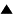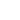# Principia Cybernetica and Principia Mathematica

## Principia Mathematica

Around the end of the last century, mathematics proposed a great variety of very successful applications: geometry, calculus, algebra, number theory, etc. Yet there was no overall theory of mathematics: these different domains functioned mainly in parallel, each with its own axioms, rules, notations, concepts, ... Most mathematicians would agree intuitively that these different subdisciplines had a "mathematical way of thinking" in common, but one had to wait for the development of mathematical logic by Boole and Frege, and set theory by Cantor before this way of thinking could be formulated more explicitly. Yet set theory and formal logic were still plagued by incoherence, paradoxes, inconsistencies and lacking connections.

One had to wait further for the classical work ofRussell andWhitehead, thePrincipia Mathematica, in which they ground the "principles of mathematical thinking" in a clear, apparently consistent and complete way. (the theorem of Gö;del later shattered the hope that such a formal treatment could ever be considered complete, but that is another story). What was novel in the work of Russell and Whitehead was that they applied mathematical methods to the foundation of mathematics itself, formulating the laws of thought governing mathematical reasoning by means of mathematical axioms, theorems and proofs. This proved highly successful, and the Principia Mathematica stills forms the basis of the "modern" mathematics as it is taught in schools and universities.

## From mathematics to cybernetics

Our contention is that something similar should be done with cybernetics: integrating and founding cybernetics with the help of cybernetical methods and tools. Similar to the mathematical application domains (number theory, geometry, etc.), the applications of cybernetics (neural networks, systems analysis, operations research, ...) need a general framework to integrate them. Similar to the integrating theories of mathematics at the end of the 19th century (Cantor's set theory, formal logic, ...), the integrating theories of cybernetics at the end of the 20th century (general systems theory, second-order cybernetics, ...) are not integrated themselves. In reference to Russell and Whitehead, the present plan for integrating them may be called the "Principia Cybernetica Project" (Turchin, 1990; Joslyn, 1990; Turchin, Joslyn and Heylighen, 1990).

## Comparing mathematics and cybernetics

Let us further indicate the similarities and differences between a Principia Mathematica and a Principia Cybernetica. Both mathematics and cybernetics are in the first place metadisciplines: they do not describe concrete objects or specific parts of the world; they describe abstract structures and processes that can be used to understand and model the world. In other words they consist of models about how to build and use models: metamodels (Van Gigh, 1986). This meta-theoretical point of view is emphasized in particular in the so-called "second order cybernetics" (von Foerster, 1979; 1981), which studies how observers construct models.

It is because of this metatheoretic character that mathematics and cybernetics can be applied to themselves: a metamodel is still a model, and hence it can be modelled by other metamodels, including itself (Heylighen, 1988). In mathematics, the most well-known example of such a self-representation is the technique of "Gödelization", where a proposition about natural numbers is represented by a natural number. Of course, it is well-known that any self-representation must be incomplete (LÖfgren, 1990), as illustrated by the Gödel theorem, but we do not consider completeness in the formal sense to be a necessary condition for a practically functioning modelling framework.

Let us proceed with the differences between cybernetics and mathematics. Mathematics is characterized by the following assumptions: simplicity, regularity and invariance; the separability of systems into independent elements; and the objective, context-independent, value-free character of knowledge. Cybernetics, on the other hand, emphasizes complexity, variety and process; the fact that elements always interact; and the subjective, context- and value-dependent nature of knowledge. Cybernetics does not deny the value of mathematics; it assumes it but goes beyond it, by trying to encompass phenomena which cannot be represented in a static, unambiguous, formal framework. It is clear then that the self-application of cybernetics, in the form of a Principia Cybernetica, must be different from the Principia Mathematica model. A Principia Cybernetica must put the emphasis on evolution and open-endedness, on different levels of precision or vagueness, on dynamic interactions between a variety of systems or viewpoints.

Part of the reason why the General Systems movement in the fifties and sixties did not succeed in its objectives was because its models and methods were still too dependent on the static, atomistic paradigm that gave birth to mathematics and classical mechanics. The reason why the present situation is much more promising is that now we dispose of better concepts, tools (e.g. computers), and methods for modelling complex and dynamic phenomena (Heylighen, 1989).

## Principles of cybernetics

Yet the idea of developing general principles (Principia) still assumes a striving towards clarity, "objectivity", and invariance. We do not want to get trapped in endless discussions and confusions over subjective meanings and viewpoints. The invariant principles that are to be derived, however, will be situated at such a high level of abstraction that they do not impose any absolute restrictions on concrete questions. They will form an empty skeleton or framework on which a multiplicity of more concrete theories can be hung (cf. Boulding, 1956). This framework will function primarily as a heuristic tool for building models, which will not preclude any model, but which will provide guidelines for avoiding difficulties and for making models more adequate. In order to succeed in that, the framework will need to incorporate methods for concretizing its own recommendations, depending on the context of the problem. This means that, unlike mathematics, the framework should provide many intermediate levels between the abstract, precise and invariant principles and their concrete, context-dependent implementations.

 HomeIntroduction to Principia CyberneticaThe Need for Principia Cybernetica Up Prev.Next Down